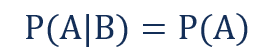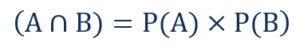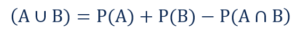# Independent Events

Two events wherein the occurrence of one event does not affect the occurrence of another event or events

## What are Independent Events?

In statistics and probability theory, independent events are two events wherein the occurrence of one event does not affect the occurrence of another event or events. The simplest example of such events is tossing two coins. The outcome of tossing the first coin cannot influence the outcome of tossing the second coin.Independent events are frequently confused with mutually exclusive events. However, they are two distinct concepts. Mutually exclusive events are events that cannot occur simultaneously. The concept of independent events is not related to the simultaneous occurrence of the events, but it is only concerned with the influence of the occurrence of one event on another.

### Independent Events and Conditional Probability

Remember that conditional probability is the probability of an event A occurring given that event B’s already occurred. If two events are independent, the probabilities of their outcomes are not dependent on each other. Therefore, the conditional probability of two independent events A and B is:The equation above may be considered as a definition of independent events. If the equation is violated, the two events are not independent.

### Probability Rules for Independent Events

Independent events follow some of the most fundamental probability rules. Some of them include:

#### 1. Rule of Multiplication

The rule of multiplication is used when we want to find the probability of events occurring simultaneously (it is also known as the joint probability of independent events). The rule of multiplication states the following:In other words, if you want to find the probability of both events A and B taking place, you should multiply the individual probabilities of the two events.

The rule of addition allows determining the probability that at least one of the events occurs (it is known as the union of events). The rule of addition is denoted:The probability of either of events A and B taking place is found by finding the sum of the individual probabilities of both events and subtracting the joint probability of the two events.

### More Resources

CFI is the official provider of the Financial Modeling and Valuation Analyst (FMVA)™ certification program, designed to transform anyone into a world-class financial analyst.

To keep learning and developing your knowledge of financial analysis, we highly recommend the additional resources below:

• Correlation
• Hypothesis Testing
• Poisson Distribution
• Quantitative Analysis

### Financial Analyst Certification

Become a certified Financial Modeling and Valuation Analyst (FMVA)® by completing CFI’s online financial modeling classes and training program!